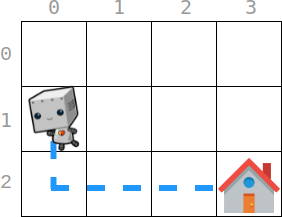Formatted question description: https://leetcode.ca/all/2087.html

# 2087. Minimum Cost Homecoming of a Robot in a Grid (Medium)

There is an m x n grid, where (0, 0) is the top-left cell and (m - 1, n - 1) is the bottom-right cell. You are given an integer array startPos where startPos = [startrow, startcol] indicates that initially, a robot is at the cell (startrow, startcol). You are also given an integer array homePos where homePos = [homerow, homecol] indicates that its home is at the cell (homerow, homecol).

The robot needs to go to its home. It can move one cell in four directions: left, right, up, or down, and it can not move outside the boundary. Every move incurs some cost. You are further given two 0-indexed integer arrays: rowCosts of length m and colCosts of length n.

• If the robot moves up or down into a cell whose row is r, then this move costs rowCosts[r].
• If the robot moves left or right into a cell whose column is c, then this move costs colCosts[c].

Return the minimum total cost for this robot to return home.

Example 1:Input: startPos = [1, 0], homePos = [2, 3], rowCosts = [5, 4, 3], colCosts = [8, 2, 6, 7]
Output: 18
Explanation: One optimal path is that:
Starting from (1, 0)
-> It goes down to (2, 0). This move costs rowCosts = 3.
-> It goes right to (2, 1). This move costs colCosts = 2.
-> It goes right to (2, 2). This move costs colCosts = 6.
-> It goes right to (2, 3). This move costs colCosts = 7.
The total cost is 3 + 2 + 6 + 7 = 18

Example 2:

Input: startPos = [0, 0], homePos = [0, 0], rowCosts = , colCosts = 
Output: 0
Explanation: The robot is already at its home. Since no moves occur, the total cost is 0.


Constraints:

• m == rowCosts.length
• n == colCosts.length
• 1 <= m, n <= 105
• 0 <= rowCosts[r], colCosts[c] <= 104
• startPos.length == 2
• homePos.length == 2
• 0 <= startrow, homerow < m
• 0 <= startcol, homecol < n

Similar Questions:

## Solution 1.

// OJ: https://leetcode.com/problems/minimum-cost-homecoming-of-a-robot-in-a-grid/
// Time: O(X + Y) where X/Y is the absolute difference between the x/y values of the startPos and homePos
// Space: O(1)
class Solution {
public:
int minCost(vector<int>& startPos, vector<int>& homePos, vector<int>& rowCosts, vector<int>& colCosts) {
int dx = homePos > startPos ? 1 : -1, dy = homePos > startPos ? 1 : -1, x = startPos, y = startPos, ans = 0;
while (x != homePos) ans += rowCosts[x += dx];
while (y != homePos) ans += colCosts[y += dy];
return ans;
}
};